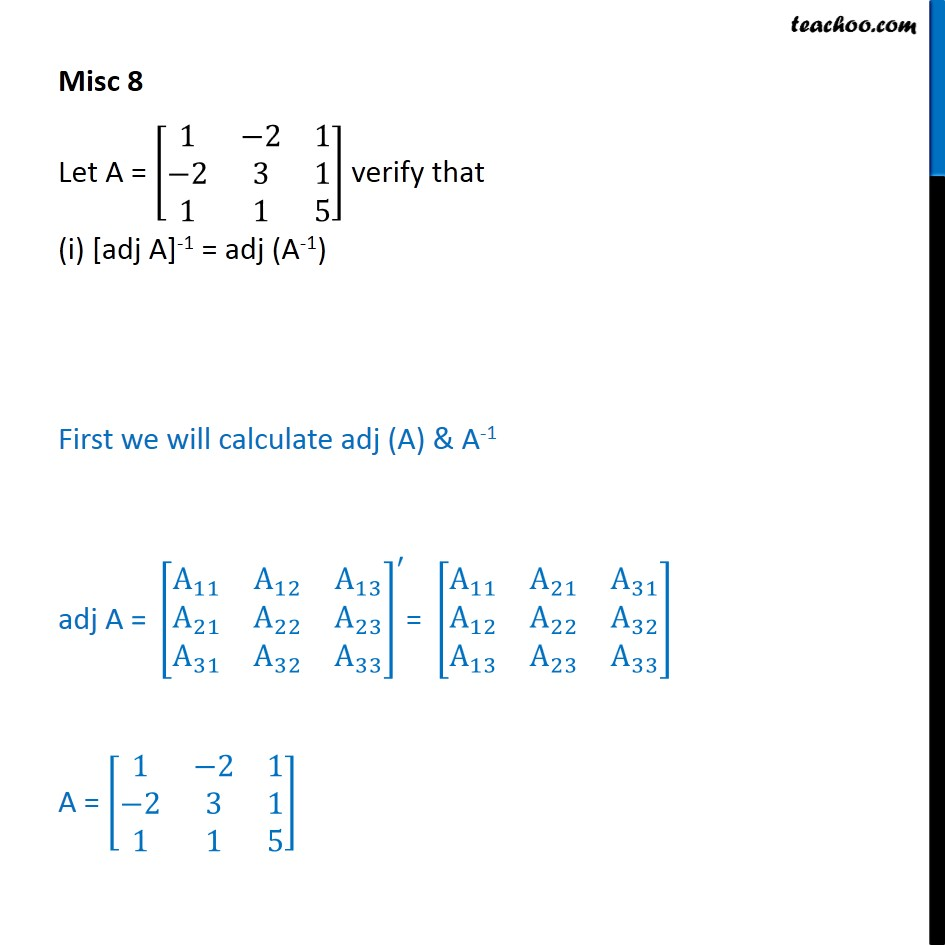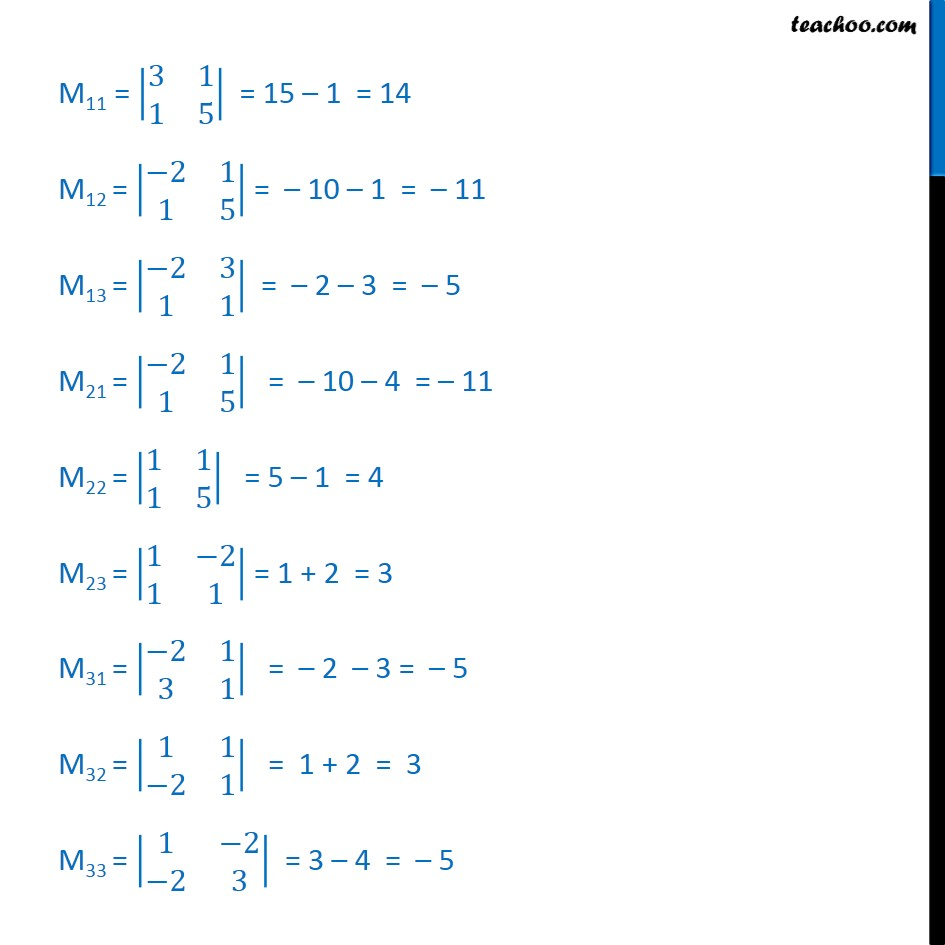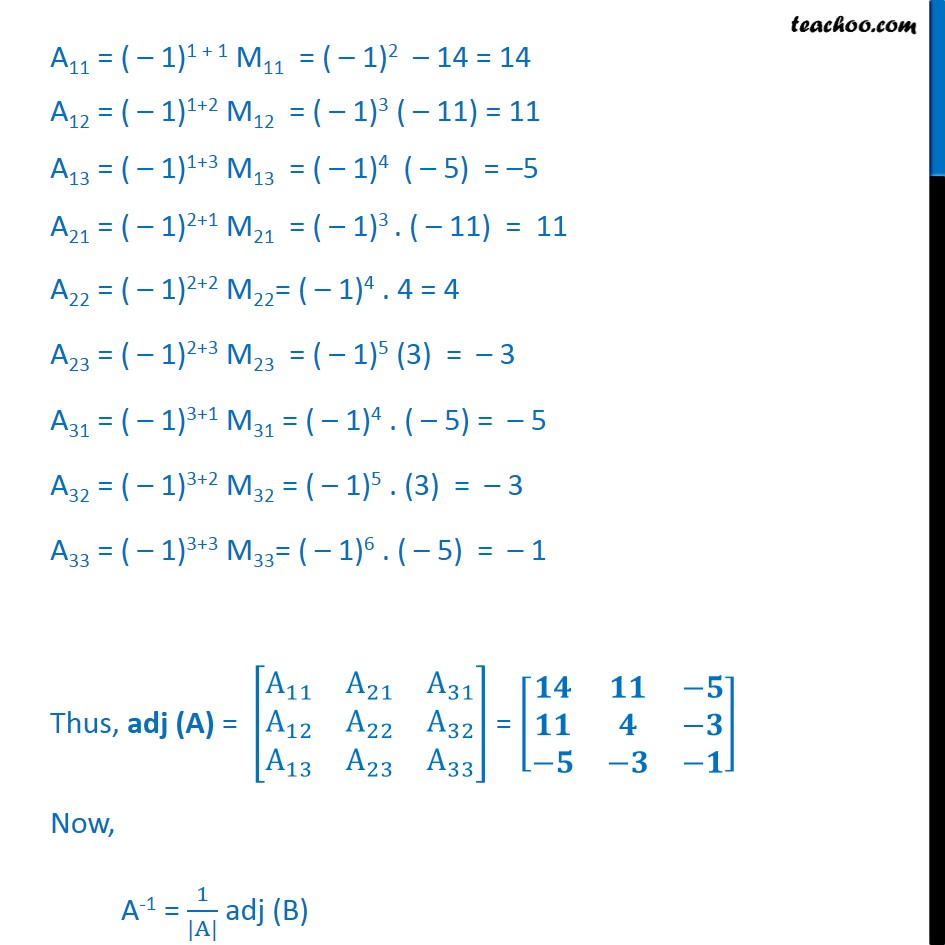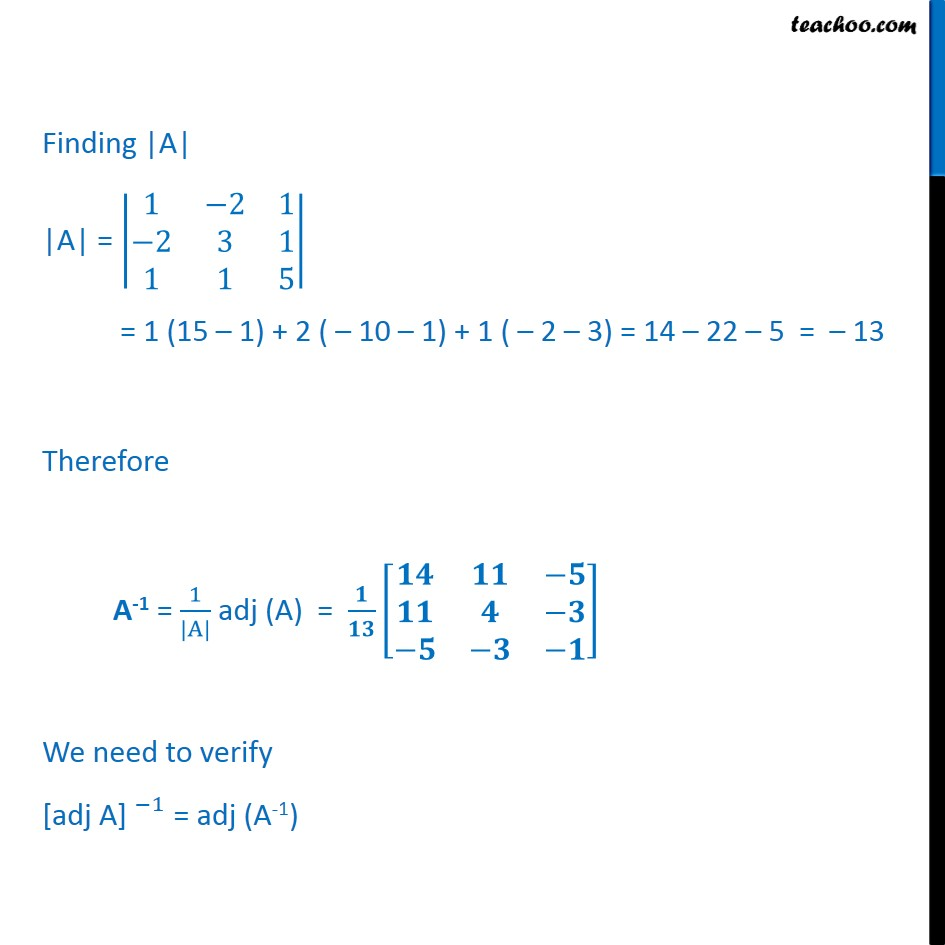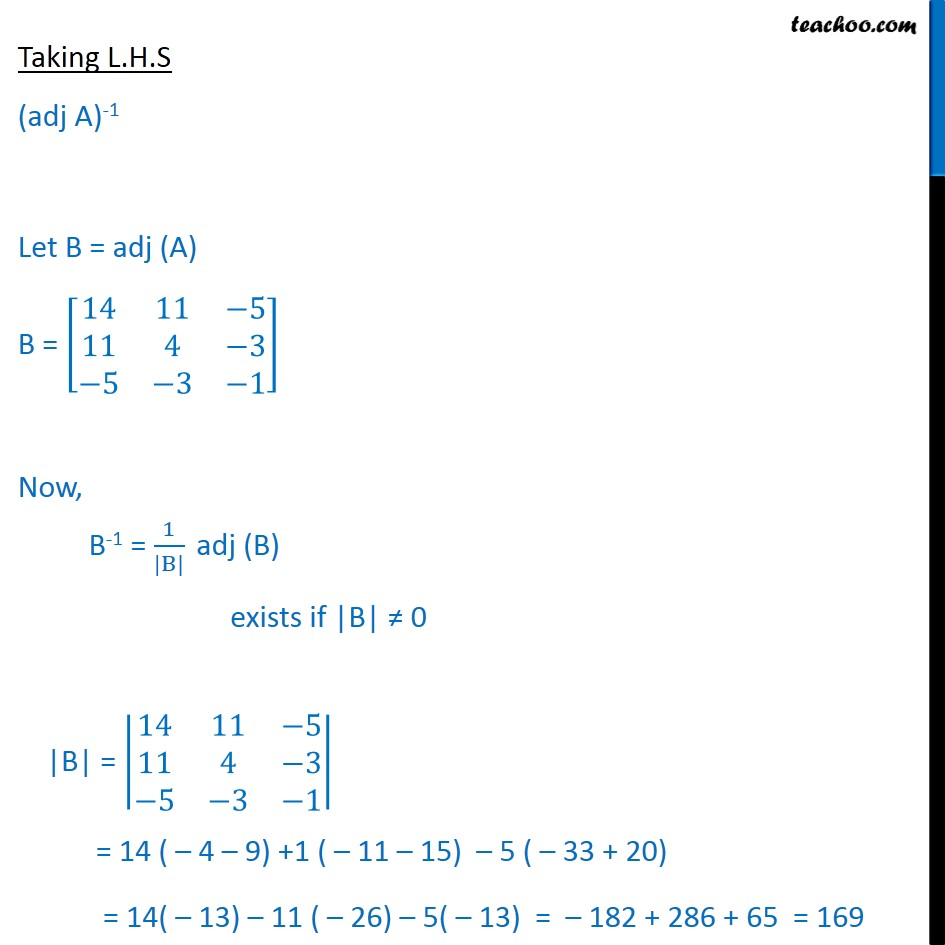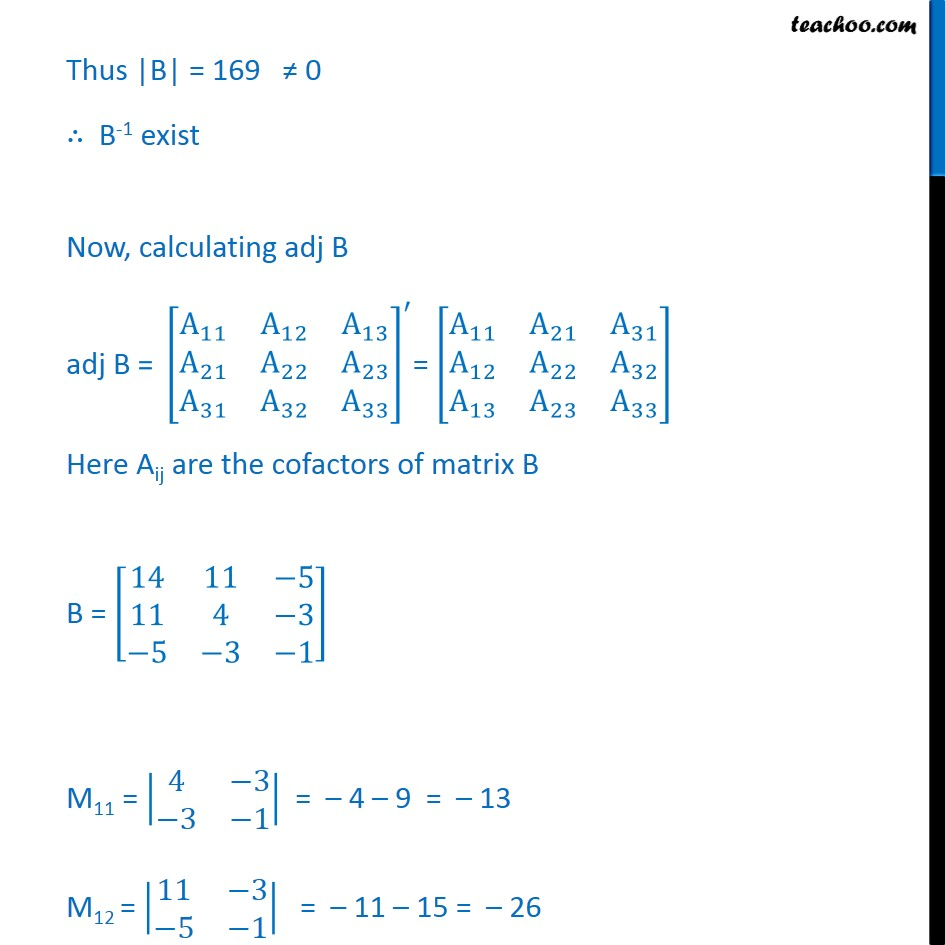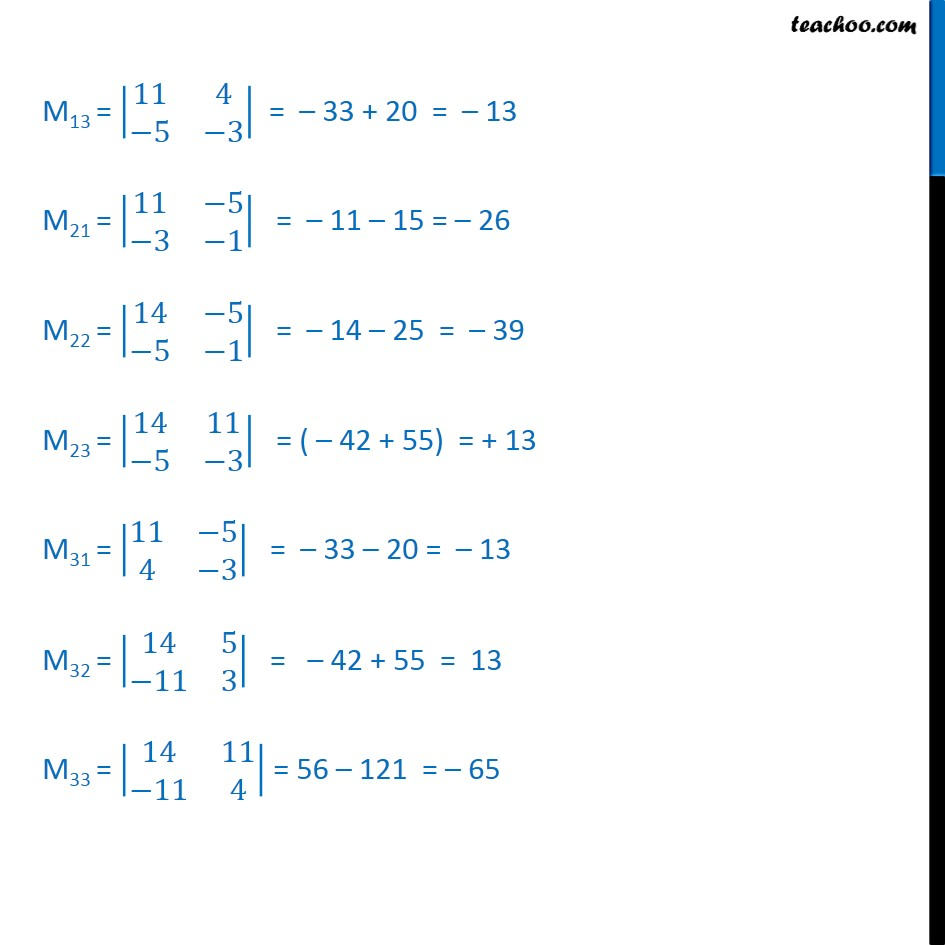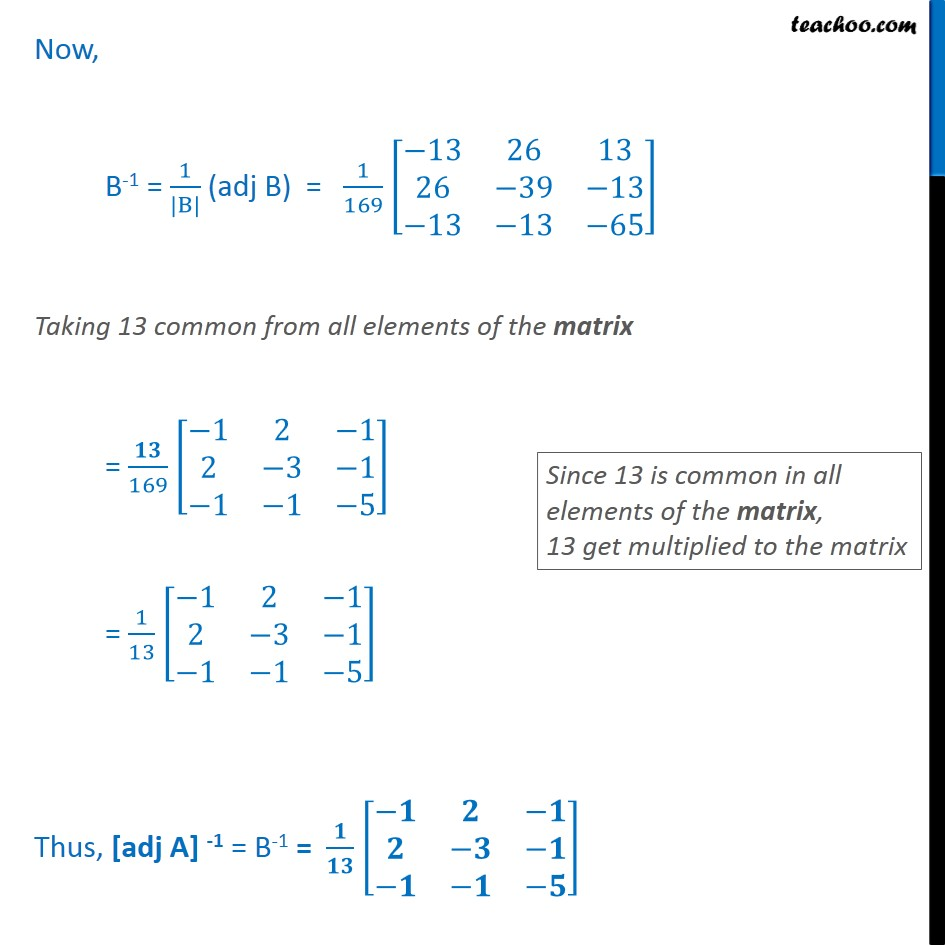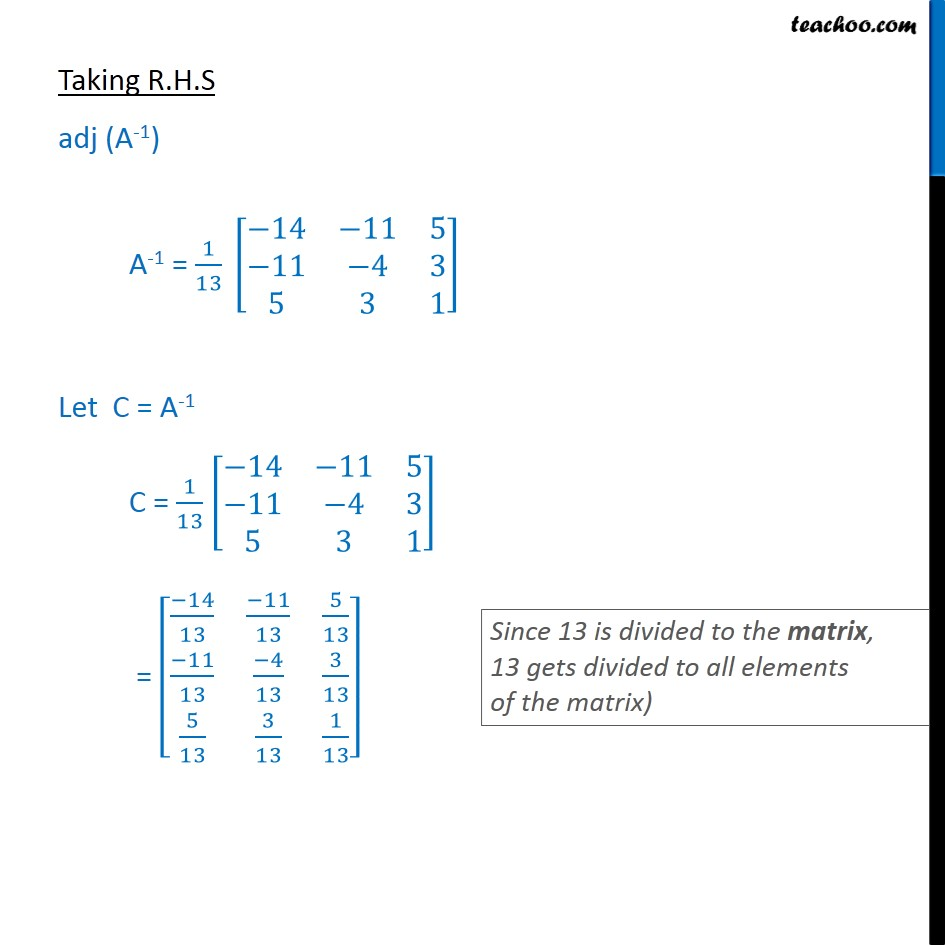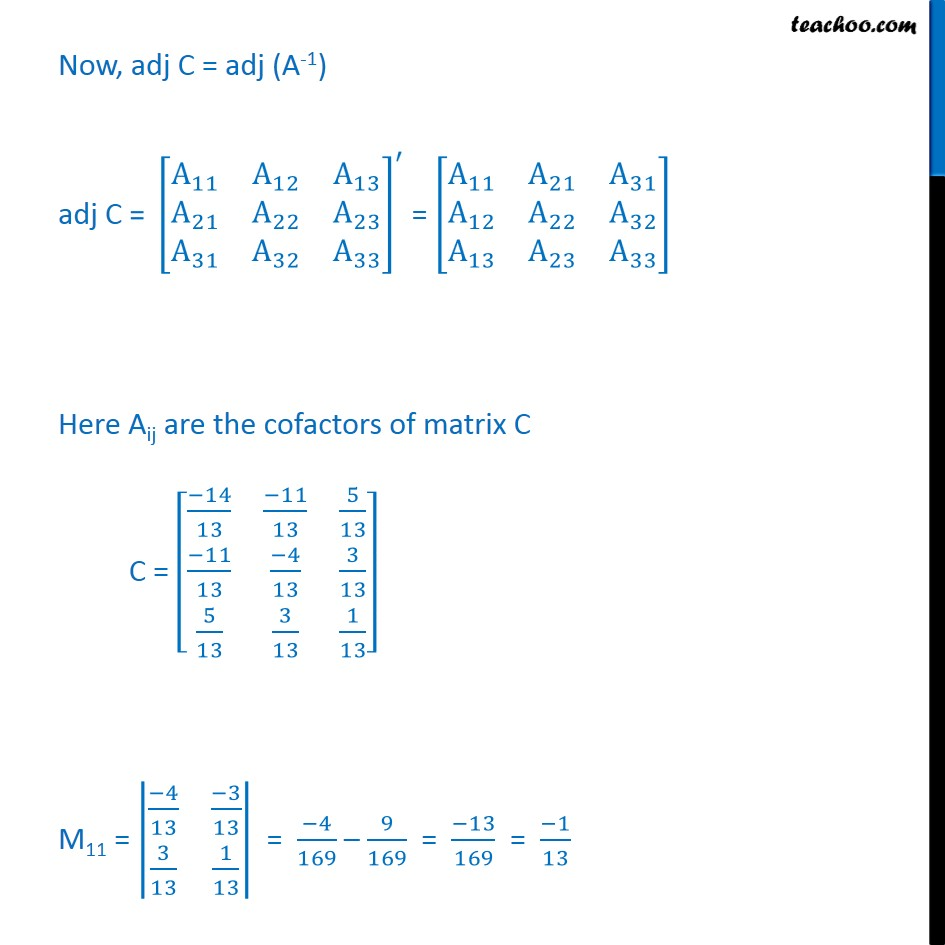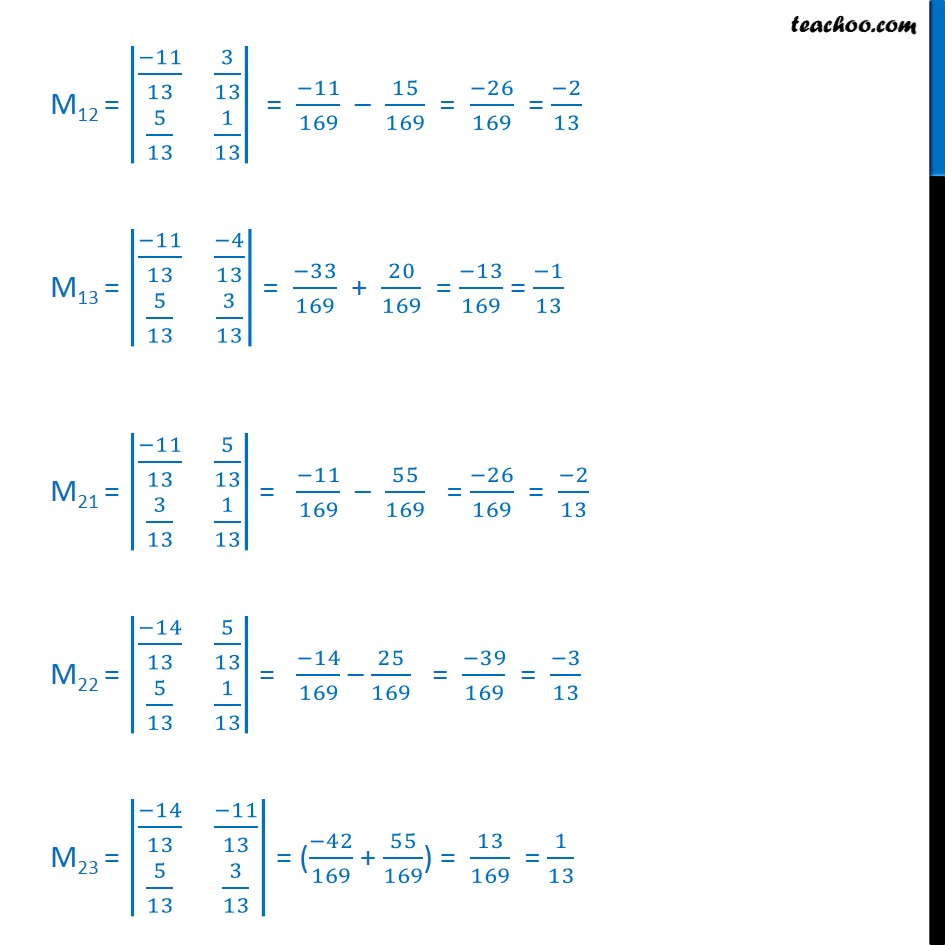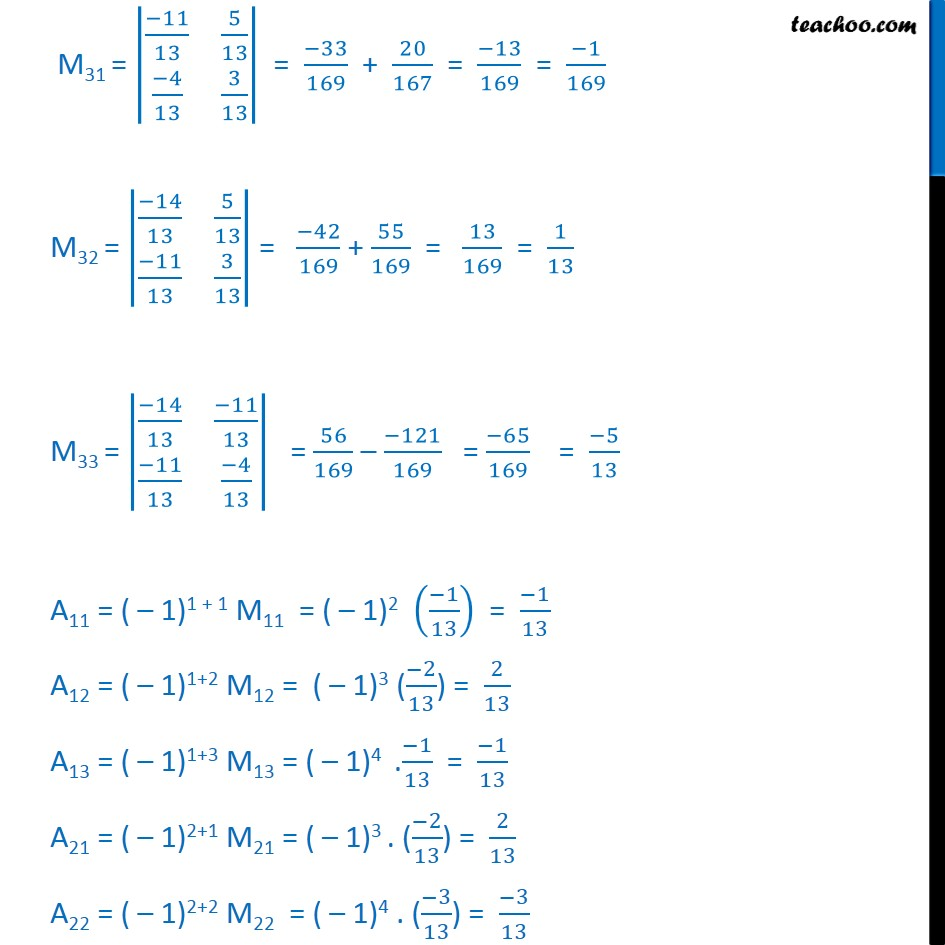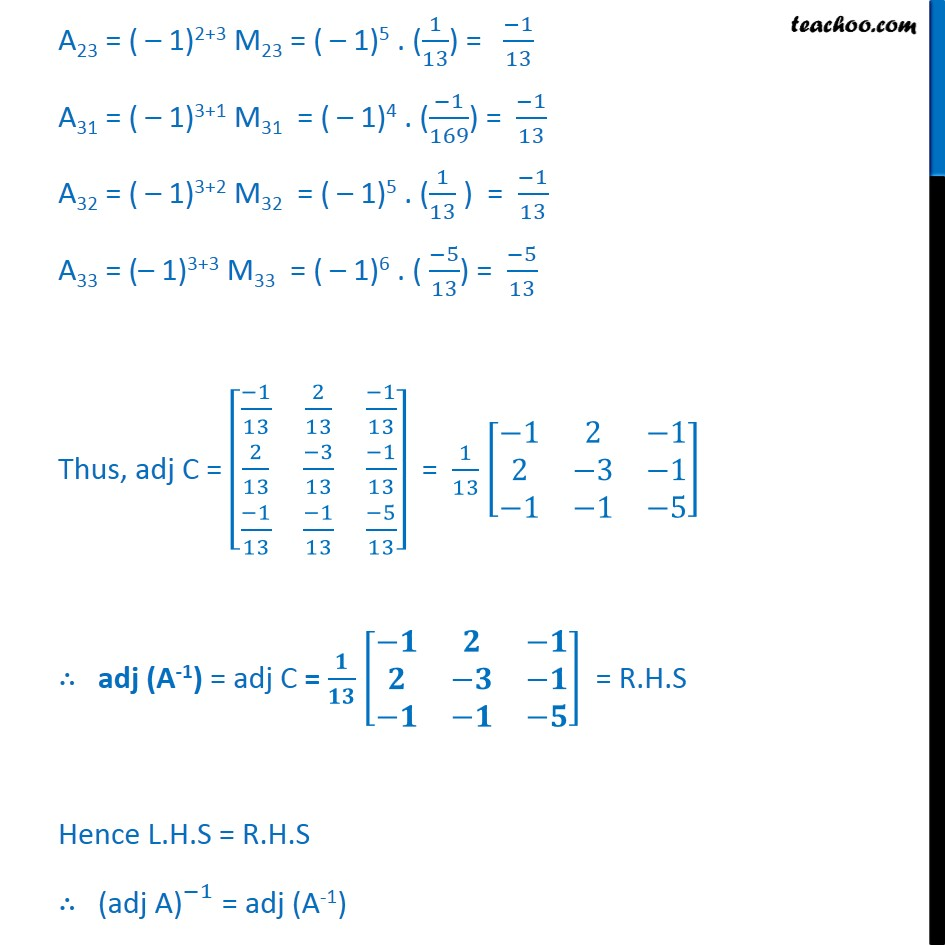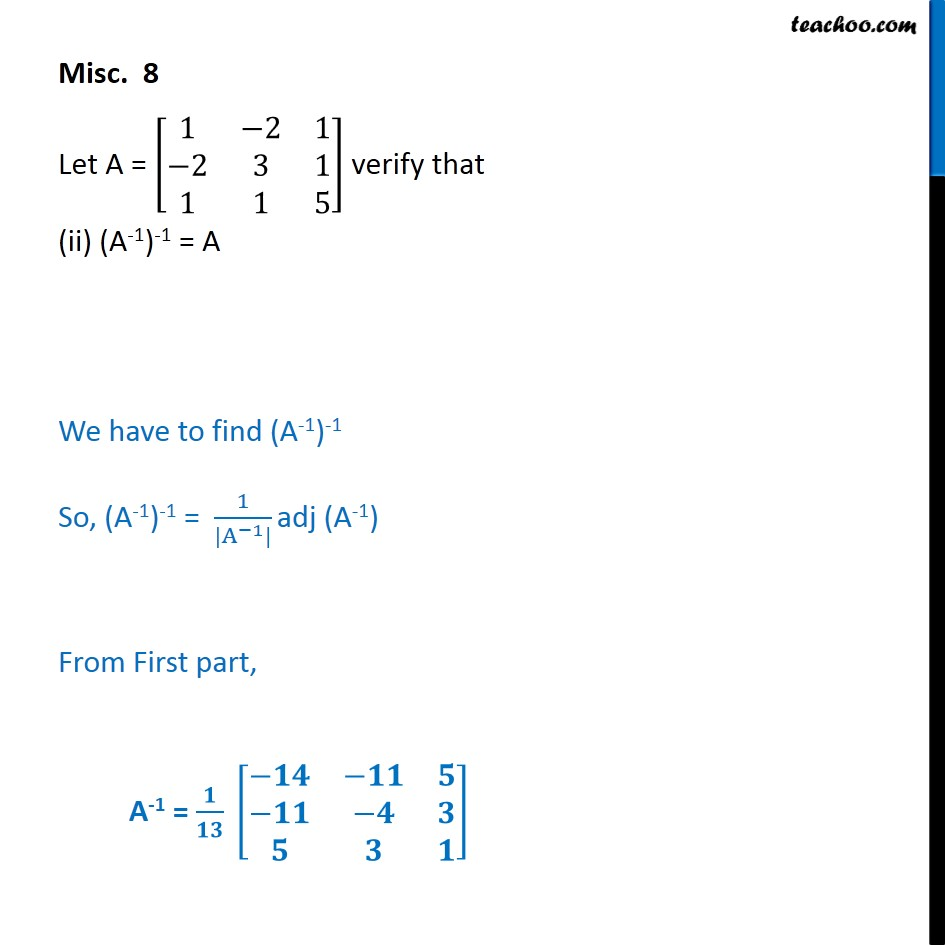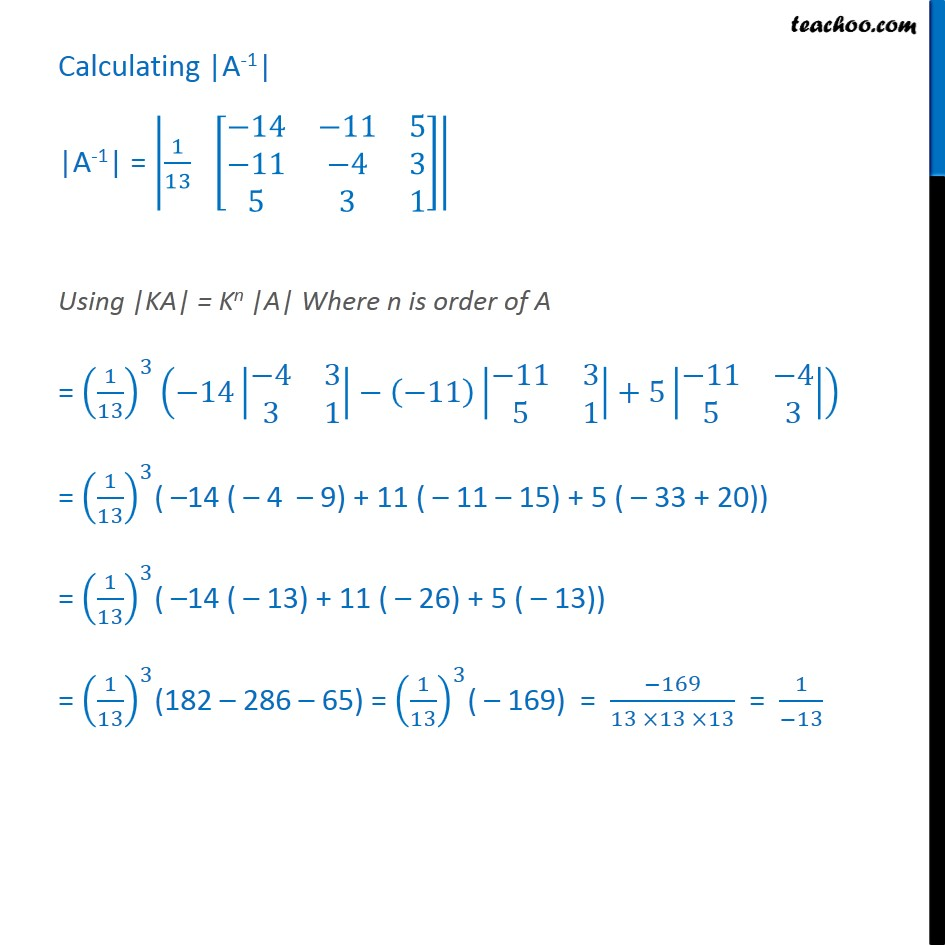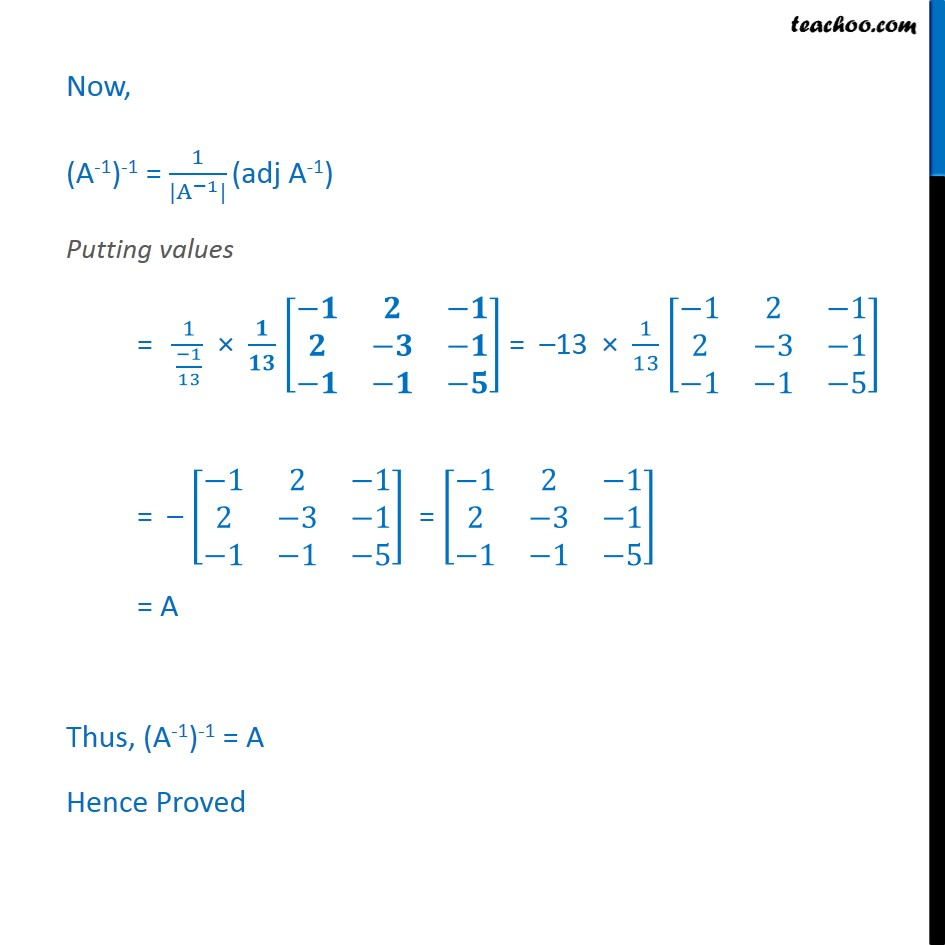1. Chapter 4 Class 12 Determinants
2. Serial order wise
3. Miscellaneous

Transcript

Misc 8 Let A = 1﷮−2﷮1﷮−2﷮3﷮1﷮1﷮1﷮5﷯﷯ verify that (i) [adj A]-1 = adj (A-1) First we will calculate adj (A) & A-1 adj A = A﷮11﷯﷮ A﷮12﷯﷮ A﷮13﷯﷮ A﷮21﷯﷮ A﷮22﷯﷮ A﷮23﷯﷮ A﷮31﷯﷮ A﷮32﷯﷮ A﷮33﷯﷯﷯﷮′﷯= A﷮11﷯﷮ A﷮21﷯﷮ A﷮31﷯﷮ A﷮12﷯﷮ A﷮22﷯﷮ A﷮32﷯﷮ A﷮13﷯﷮ A﷮23﷯﷮ A﷮33﷯﷯﷯ A = 1﷮−2﷮1﷮−2﷮3﷮1﷮1﷮1﷮5﷯﷯ M11 = 3﷮1﷮1﷮5﷯﷯ = 15 – 1 = 14 M12 = −2﷮1﷮1﷮5﷯﷯ = – 10 – 1 = – 11 M13 = −2﷮3﷮1﷮1﷯﷯ = – 2 – 3 = – 5 M21 = −2﷮1﷮1﷮5﷯﷯ = – 10 – 4 = – 11 M22 = 1﷮1﷮1﷮5﷯﷯ = 5 – 1 = 4 M23 = 1﷮−2﷮1﷮1﷯﷯ = 1 + 2 = 3 M31 = −2﷮1﷮3﷮1﷯﷯ = – 2 – 3 = – 5 M32 = 1﷮1﷮−2﷮1﷯﷯ = 1 + 2 = 3 M33 = 1﷮−2﷮−2﷮3﷯﷯ = 3 – 4 = – 5 A11 = ( – 1)1 + 1 M11 = ( – 1)2 – 14 = 14 A12 = ( – 1)1+2 M12 = ( – 1)3 ( – 11) = 11 A13 = ( – 1)1+3 M13 = ( – 1)4 ( – 5) = –5 A21 = ( – 1)2+1 M21 = ( – 1)3 . ( – 11) = 11 A22 = ( – 1)2+2 M22= ( – 1)4 . 4 = 4 A23 = ( – 1)2+3 M23 = ( – 1)5 (3) = – 3 A31 = ( – 1)3+1 M31 = ( – 1)4 . ( – 5) = – 5 A32 = ( – 1)3+2 M32 = ( – 1)5 . (3) = – 3 A33 = ( – 1)3+3 M33= ( – 1)6 . ( – 5) = – 1 Thus, adj (A) = A﷮11﷯﷮ A﷮21﷯﷮ A﷮31﷯﷮ A﷮12﷯﷮ A﷮22﷯﷮ A﷮32﷯﷮ A﷮13﷯﷮ A﷮23﷯﷮ A﷮33﷯﷯﷯ = 𝟏𝟒﷮𝟏𝟏﷮−𝟓﷮𝟏𝟏﷮𝟒﷮−𝟑﷮−𝟓﷮−𝟑﷮−𝟏﷯﷯ Now, A-1 = 1﷮|A|﷯ adj (B) Finding |A| |A| = 1﷮−2﷮1﷮−2﷮3﷮1﷮1﷮1﷮5﷯﷯ = 1 (15 – 1) + 2 ( – 10 – 1) + 1 ( – 2 – 3) = 14 – 22 – 5 = – 13 Therefore A-1 = 1﷮|A|﷯ adj (A) = 𝟏﷮𝟏𝟑﷯ 𝟏𝟒﷮𝟏𝟏﷮−𝟓﷮𝟏𝟏﷮𝟒﷮−𝟑﷮−𝟓﷮−𝟑﷮−𝟏﷯﷯ We need to verify [adj A] ﷮−1﷯ = adj (A-1) Taking L.H.S (adj A)-1 Let B = adj (A) B = 14﷮11﷮−5﷮11﷮4﷮−3﷮−5﷮−3﷮−1﷯﷯ Now, B-1 = 1﷮|B|﷯ adj (B) exists if |B| ≠ 0 |B| = 14﷮11﷮−5﷮11﷮4﷮−3﷮−5﷮−3﷮−1﷯﷯ = 14 ( – 4 – 9) +1 ( – 11 – 15) – 5 ( – 33 + 20) = 14( – 13) – 11 ( – 26) – 5( – 13) = – 182 + 286 + 65 = 169 Thus |B| = 169 ≠ 0 ∴ B-1 exist Now, calculating adj B adj B = A﷮11﷯﷮ A﷮12﷯﷮ A﷮13﷯﷮ A﷮21﷯﷮ A﷮22﷯﷮ A﷮23﷯﷮ A﷮31﷯﷮ A﷮32﷯﷮ A﷮33﷯﷯﷯﷮′﷯= A﷮11﷯﷮ A﷮21﷯﷮ A﷮31﷯﷮ A﷮12﷯﷮ A﷮22﷯﷮ A﷮32﷯﷮ A﷮13﷯﷮ A﷮23﷯﷮ A﷮33﷯﷯﷯ Here Aij are the cofactors of matrix B B = 14﷮11﷮−5﷮11﷮4﷮−3﷮−5﷮−3﷮−1﷯﷯ M11 = 4﷮−3﷮−3﷮−1﷯﷯ = – 4 – 9 = – 13 M12 = 11﷮−3﷮−5﷮−1﷯﷯ = – 11 – 15 = – 26 M13 = 11﷮4﷮−5﷮−3﷯﷯ = – 33 + 20 = – 13 M21 = 11﷮−5﷮−3﷮−1﷯﷯ = – 11 – 15 = – 26 M22 = 14﷮−5﷮−5﷮−1﷯﷯ = – 14 – 25 = – 39 M23 = 14﷮11﷮−5﷮−3﷯﷯ = ( – 42 + 55) = + 13 M31 = 11﷮−5﷮4﷮−3﷯﷯ = – 33 – 20 = – 13 M32 = 14﷮5﷮−11﷮3﷯﷯ = – 42 + 55 = 13 M33 = 14﷮11﷮−11﷮4﷯﷯ = 56 – 121 = – 65 A11 = ( – 1)1 + 1 M11 = ( – 1)2 (– 13) = – 13 A12 = ( – 1)1+2 M12 = ( – 1)3 ( – 26) = 26 A13 = ( – 1)1+3 M13 = ( – 1)4 . ( – 13) = – 13 A21 = ( – 1)2+1 M21 = ( – 1)3 . ( – 26) = 26 A22 = ( – 1)2+2 M22= ( – 1)4 . ( – 39) = – 39 A23 = ( – 1)2+3 M23 = ( – 1)5 . ( – 13) = – 13 A31 = ( – 1)3+1 M31 = ( – 1)4 . ( – 13) = – 13 A32 = ( – 1)3+2 M32 = ( – 1)5 . (13) = – 13 A33 = ( – 1)3+3 M33 = ( – 1)6 . ( – 65) = – 65 Thus, adj B = A﷮11﷯﷮ A﷮21﷯﷮ A﷮31﷯﷮ A﷮12﷯﷮ A﷮22﷯﷮ A﷮32﷯﷮ A﷮13﷯﷮ A﷮23﷯﷮ A﷮33﷯﷯﷯ = −13﷮26﷮13﷮26﷮−39﷮−13﷮−13﷮−13﷮−65﷯﷯ Now, B-1 = 1﷮|B|﷯ (adj B) = 1﷮169﷯ −13﷮26﷮13﷮26﷮−39﷮−13﷮−13﷮−13﷮−65﷯﷯ Taking 13 common from all elements of the matrix = 𝟏𝟑﷮169﷯ −1﷮2﷮−1﷮2﷮−3﷮−1﷮−1﷮−1﷮−5﷯﷯ = 1﷮13﷯ −1﷮2﷮−1﷮2﷮−3﷮−1﷮−1﷮−1﷮−5﷯﷯ Thus, [adj A] -1 = B-1 = 𝟏﷮𝟏𝟑﷯ −𝟏﷮𝟐﷮−𝟏﷮𝟐﷮−𝟑﷮−𝟏﷮−𝟏﷮−𝟏﷮−𝟓﷯﷯ Taking R.H.S adj (A-1) A-1 = 1﷮13﷯ −14﷮−11﷮5﷮−11﷮−4﷮3﷮5﷮3﷮1﷯﷯ Let C = A-1 C = 1﷮13﷯ −14﷮−11﷮5﷮−11﷮−4﷮3﷮5﷮3﷮1﷯﷯ = −14﷮13﷯﷮ −11﷮13﷯﷮ 5﷮13﷯﷮ −11﷮13﷯﷮ −4﷮13﷯﷮ 3﷮13﷯﷮ 5﷮13﷯﷮ 3﷮13﷯﷮ 1﷮13﷯﷯﷯ Now, adj C = adj (A-1) adj C = A﷮11﷯﷮ A﷮12﷯﷮ A﷮13﷯﷮ A﷮21﷯﷮ A﷮22﷯﷮ A﷮23﷯﷮ A﷮31﷯﷮ A﷮32﷯﷮ A﷮33﷯﷯﷯﷮′﷯ = A﷮11﷯﷮ A﷮21﷯﷮ A﷮31﷯﷮ A﷮12﷯﷮ A﷮22﷯﷮ A﷮32﷯﷮ A﷮13﷯﷮ A﷮23﷯﷮ A﷮33﷯﷯﷯ Here Aij are the cofactors of matrix C C = −14﷮13﷯﷮ −11﷮13﷯﷮ 5﷮13﷯﷮ −11﷮13﷯﷮ −4﷮13﷯﷮ 3﷮13﷯﷮ 5﷮13﷯﷮ 3﷮13﷯﷮ 1﷮13﷯﷯﷯ M11 = −4﷮13﷯﷮ −3﷮13﷯﷮ 3﷮13﷯﷮ 1﷮13﷯﷯﷯ = −4﷮169﷯ – 9﷮169﷯ = −13﷮169﷯ = −1﷮13﷯ M12 = −11﷮13﷯﷮ 3﷮13﷯﷮ 5﷮13﷯﷮ 1﷮13﷯﷯﷯ = −11﷮169﷯ – 15﷮169﷯ = −26﷮169﷯ = −2﷮13﷯ M13 = −11﷮13﷯﷮ −4﷮13﷯﷮ 5﷮13﷯﷮ 3﷮13﷯﷯﷯ = −33﷮169﷯ + 20﷮169﷯ = −13﷮169﷯ = −1﷮13﷯ M21 = −11﷮13﷯﷮ 5﷮13﷯﷮ 3﷮13﷯﷮ 1﷮13﷯﷯﷯ = −11﷮169﷯ – 55﷮169﷯ = −26﷮169﷯ = −2﷮13﷯ M22 = −14﷮13﷯﷮ 5﷮13﷯﷮ 5﷮13﷯﷮ 1﷮13﷯﷯﷯ = −14﷮169﷯ – 25﷮169﷯ = −39﷮169﷯ = −3﷮13﷯ M23 = −14﷮13﷯﷮ −11﷮13﷯﷮ 5﷮13﷯﷮ 3﷮13﷯﷯﷯ = ( −42﷮169﷯ + 55﷮169﷯) = 13﷮169﷯ = 1﷮13﷯ M31 = −11﷮13﷯﷮ 5﷮13﷯﷮ −4﷮13﷯﷮ 3﷮13﷯﷯﷯ = −33﷮169﷯ + 20﷮167﷯ = −13﷮169﷯ = −1﷮169﷯ M32 = −14﷮13﷯﷮ 5﷮13﷯﷮ −11﷮13﷯﷮ 3﷮13﷯﷯﷯ = −42﷮169﷯ + 55﷮169﷯ = 13﷮169﷯ = 1﷮13﷯ M33 = −14﷮13﷯﷮ −11﷮13﷯﷮ −11﷮13﷯﷮ −4﷮13﷯﷯﷯ = 56﷮169﷯ – −121﷮169﷯ = −65﷮169﷯ = −5﷮13﷯ A11 = ( – 1)1 + 1 M11 = ( – 1)2 −1﷮13﷯﷯ = −1﷮13﷯ A12 = ( – 1)1+2 M12 = ( – 1)3 ( −2﷮13﷯) = 2﷮13﷯ A13 = ( – 1)1+3 M13 = ( – 1)4 . −1﷮13﷯ = −1﷮13﷯ A21 = ( – 1)2+1 M21 = ( – 1)3 . ( −2﷮13﷯) = 2﷮13﷯ A22 = ( – 1)2+2 M22 = ( – 1)4 . ( −3﷮13﷯) = −3﷮13﷯ A23 = ( – 1)2+3 M23 = ( – 1)5 . ( 1﷮13﷯) = −1﷮13﷯ A31 = ( – 1)3+1 M31 = ( – 1)4 . ( −1﷮169﷯) = −1﷮13﷯ A32 = ( – 1)3+2 M32 = ( – 1)5 . ( 1﷮13﷯ ) = −1﷮13﷯ A33 = (– 1)3+3 M33 = ( – 1)6 . ( −5﷮13﷯) = −5﷮13﷯ Thus, adj C = −1﷮13﷯﷮ 2﷮13﷯﷮ −1﷮13﷯﷮ 2﷮13﷯﷮ −3﷮13﷯﷮ −1﷮13﷯﷮ −1﷮13﷯﷮ −1﷮13﷯﷮ −5﷮13﷯﷯﷯ = 1﷮13﷯ −1﷮2﷮−1﷮2﷮−3﷮−1﷮−1﷮−1﷮−5﷯﷯ ∴ adj (A-1) = adj C = 𝟏﷮𝟏𝟑﷯ −𝟏﷮𝟐﷮−𝟏﷮𝟐﷮−𝟑﷮−𝟏﷮−𝟏﷮−𝟏﷮−𝟓﷯﷯ = R.H.S Hence L.H.S = R.H.S ∴ (adj A)﷮−1﷯ = adj (A-1) Misc. 8 Let A = 1﷮−2﷮1﷮−2﷮3﷮1﷮1﷮1﷮5﷯﷯ verify that (ii) (A-1)-1 = A We have to find (A-1)-1 So, (A-1)-1 = 1﷮| A﷮−1﷯|﷯ adj (A-1) From First part, A-1 = 𝟏﷮𝟏𝟑﷯ −𝟏𝟒﷮−𝟏𝟏﷮𝟓﷮−𝟏𝟏﷮−𝟒﷮𝟑﷮𝟓﷮𝟑﷮𝟏﷯﷯ Calculating |A-1| |A-1| = 1﷮13﷯ −14﷮−11﷮5﷮−11﷮−4﷮3﷮5﷮3﷮1﷯﷯﷯ Using |KA| = Kn |A| Where n is order of A = 1﷮13﷯﷯﷮3﷯ −14 −4﷮3﷮3﷮1﷯﷯− −11﷯ −11﷮3﷮5﷮1﷯﷯+5 −11﷮−4﷮5﷮3﷯﷯﷯ = 1﷮13﷯﷯﷮3﷯( –14 ( – 4 – 9) + 11 ( – 11 – 15) + 5 ( – 33 + 20)) = 1﷮13﷯﷯﷮3﷯( –14 ( – 13) + 11 ( – 26) + 5 ( – 13)) = 1﷮13﷯﷯﷮3﷯(182 – 286 – 65) = 1﷮13﷯﷯﷮3﷯( – 169) = −169﷮13 ×13 ×13﷯ = 1﷮−13﷯ Now, (A-1)-1 = 1﷮| A﷮−1﷯|﷯ (adj A-1) Putting values = 1﷮ −1﷮13﷯﷯ × 𝟏﷮𝟏𝟑﷯ −𝟏﷮𝟐﷮−𝟏﷮𝟐﷮−𝟑﷮−𝟏﷮−𝟏﷮−𝟏﷮−𝟓﷯﷯ = –13 × 1﷮13﷯ −1﷮2﷮−1﷮2﷮−3﷮−1﷮−1﷮−1﷮−5﷯﷯ = – −1﷮2﷮−1﷮2﷮−3﷮−1﷮−1﷮−1﷮−5﷯﷯ = −1﷮2﷮−1﷮2﷮−3﷮−1﷮−1﷮−1﷮−5﷯﷯ = A Thus, (A-1)-1 = A Hence Proved Now, (A-1)-1 = 1﷮| A﷮−1﷯|﷯ (adj A-1) Putting values = 1﷮ −1﷮13﷯﷯ × 𝟏﷮𝟏𝟑﷯ −𝟏﷮𝟐﷮−𝟏﷮𝟐﷮−𝟑﷮−𝟏﷮−𝟏﷮−𝟏﷮−𝟓﷯﷯ = –13 × 1﷮13﷯ −1﷮2﷮−1﷮2﷮−3﷮−1﷮−1﷮−1﷮−5﷯﷯ = – −1﷮2﷮−1﷮2﷮−3﷮−1﷮−1﷮−1﷮−5﷯﷯ = −1﷮2﷮−1﷮2﷮−3﷮−1﷮−1﷮−1﷮−5﷯﷯ = A Thus, (A-1)-1 = A Hence Proved

Miscellaneous

About the AuthorDavneet Singh
Davneet Singh is a graduate from Indian Institute of Technology, Kanpur. He has been teaching from the past 9 years. He provides courses for Maths and Science at Teachoo.